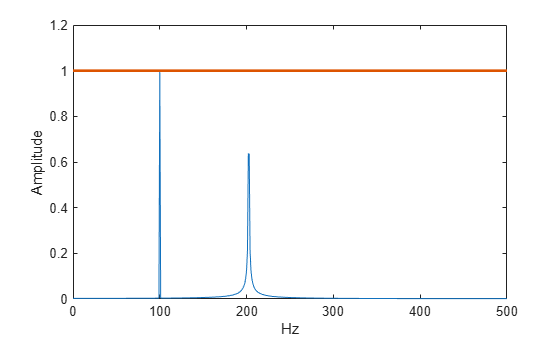# Amplitude Estimation and Zero Padding

This example shows how to use zero padding to obtain an accurate estimate of the amplitude of a sinusoidal signal. Frequencies in the discrete Fourier transform (DFT) are spaced at intervals of ${F}_{s}/N$, where ${F}_{s}$ is the sample rate and $N$ is the length of the input time series. Attempting to estimate the amplitude of a sinusoid with a frequency that does not correspond to a DFT bin can result in an inaccurate estimate. Zero padding the data before computing the DFT often helps to improve the accuracy of amplitude estimates.

Create a signal consisting of two sine waves. The two sine waves have frequencies of 100 and 202.5 Hz. The sample rate is 1000 Hz and the signal is 1000 samples in length.

```Fs = 1e3; t = 0:0.001:1-0.001; x = cos(2*pi*100*t)+sin(2*pi*202.5*t);```

Obtain the DFT of the signal. The DFT bins are spaced at 1 Hz. Accordingly, the 100 Hz sine wave corresponds to a DFT bin, but the 202.5 Hz sine wave does not.

Because the signal is real-valued, use only the positive frequencies from the DFT to estimate the amplitude. Scale the DFT by the length of the input signal and multiply all frequencies except 0 and the Nyquist by 2.

Plot the result with the known amplitudes for comparison.

```xdft = fft(x); xdft = xdft(1:length(x)/2+1); xdft = xdft/length(x); xdft(2:end-1) = 2*xdft(2:end-1); freq = 0:Fs/length(x):Fs/2; plot(freq,abs(xdft)) hold on plot(freq,ones(length(x)/2+1,1),'LineWidth',2) xlabel('Hz') ylabel('Amplitude') hold off```The amplitude estimate at 100 Hz is accurate because that frequency corresponds to a DFT bin. However, the amplitude estimate at 202.5 Hz is not accurate because that frequency does not correspond to a DFT bin.

You can interpolate the DFT by zero padding. Zero padding enables you to obtain more accurate amplitude estimates of resolvable signal components. On the other hand, zero padding does not improve the spectral (frequency) resolution of the DFT. The resolution is determined by the number of samples and the sample rate.

Pad the DFT out to 2000, or twice the original length of `x`. With this length, the spacing between DFT bins is ${F}_{s}/2000=0.5\phantom{\rule{0.2777777777777778em}{0ex}}Hz$. In this case, the energy from the 202.5 Hz sine wave falls directly in a DFT bin. Obtain the DFT and plot the amplitude estimates. Use zero padding out to 2000 samples.

```lpad = 2*length(x); xdft = fft(x,lpad); xdft = xdft(1:lpad/2+1); xdft = xdft/length(x); xdft(2:end-1) = 2*xdft(2:end-1); freq = 0:Fs/lpad:Fs/2; plot(freq,abs(xdft)) hold on plot(freq,ones(2*length(x)/2+1,1),'LineWidth',2) xlabel('Hz') ylabel('Amplitude') hold off```The use of zero padding enables you to estimate the amplitudes of both frequencies correctly.